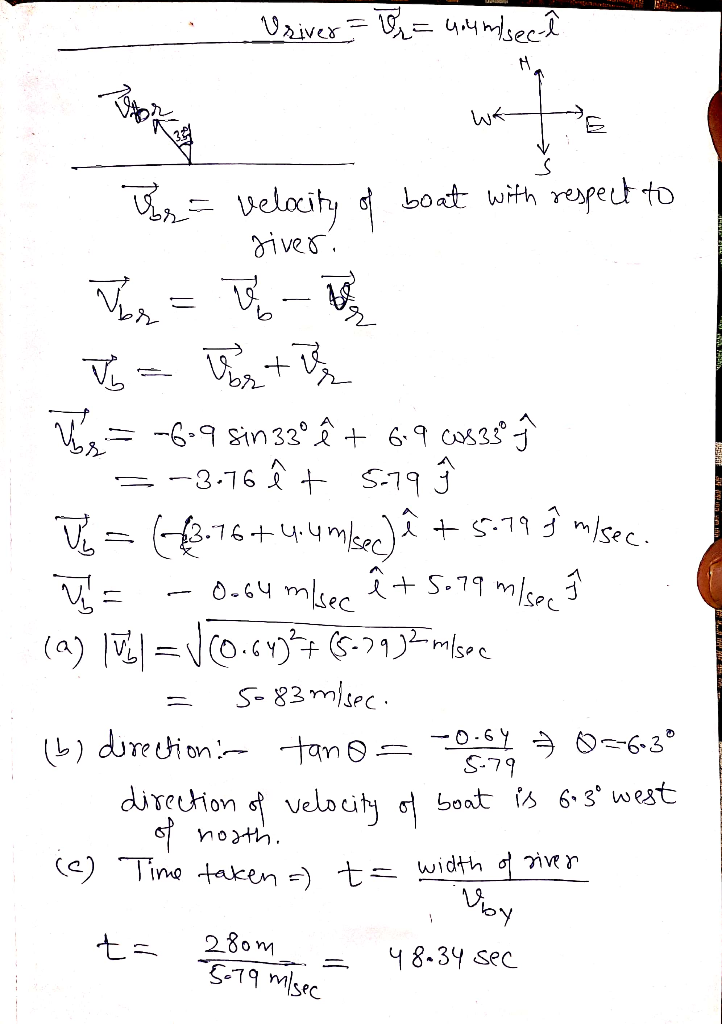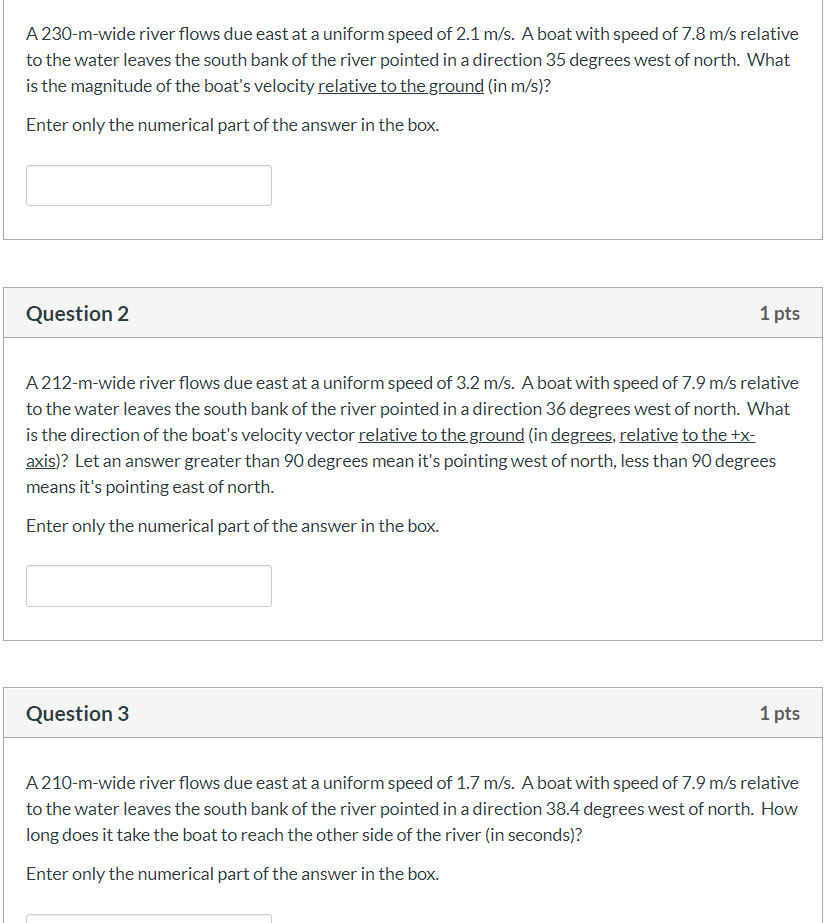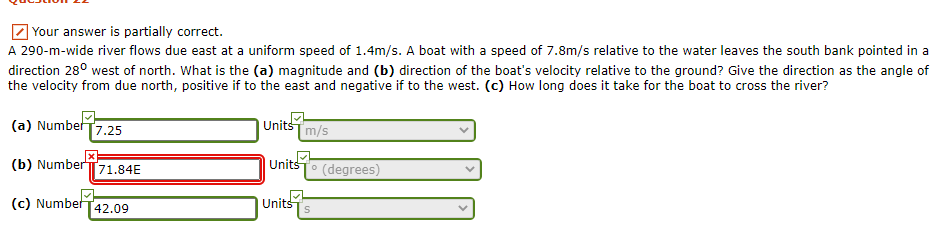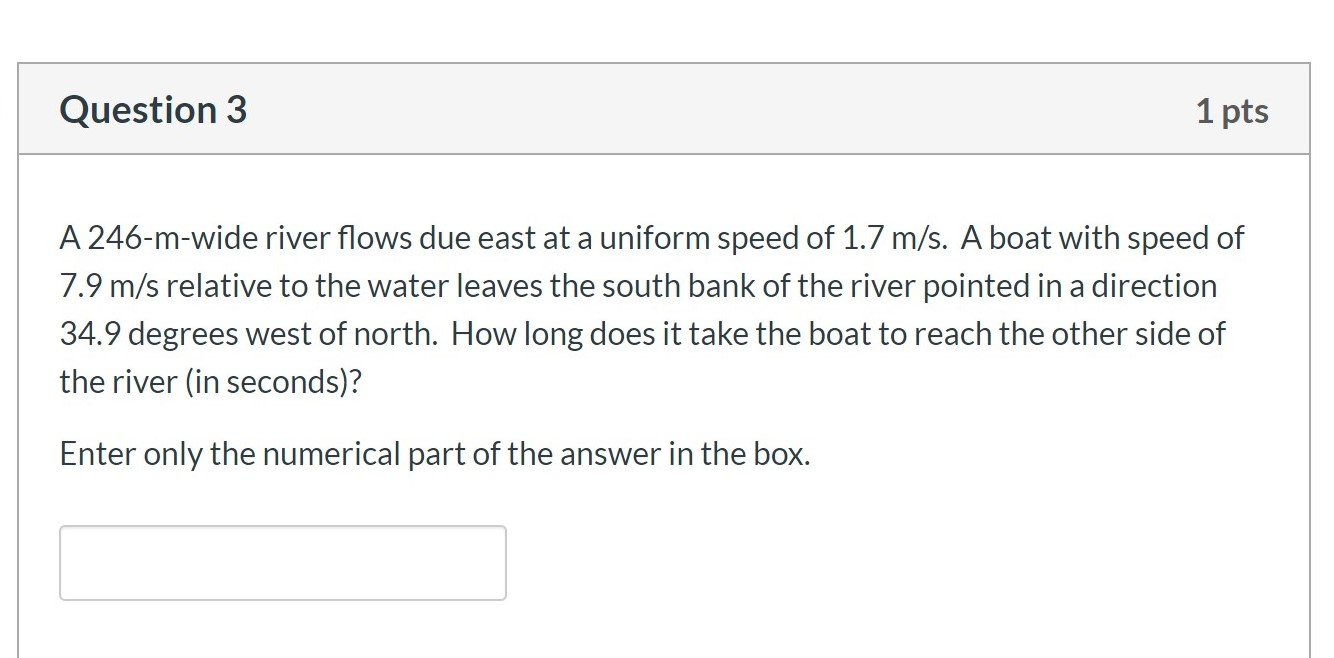Question

A 280-m-wide river flows due east at a uniform speed of 4.4m/s. A boat with a speed of 6.9m/s relative to the water leaves the south bank pointed in a direction 33owest of north. What is the (a) magnitude and (b)direction of the boat's velocity relative to the ground? Give the direction as the angle of the velocity from due north, positive if to the east and negative if to the west. (c) How long does it take for the boat to cross the river?#### Earn Coins

Coins can be redeemed for fabulous gifts.

Similar Homework Help Questions
• ### A 200-m-wide river flows due east at a uniform speed of 3.4m/s. A boat with a...

A 200-m-wide river flows due east at a uniform speed of 3.4m/s. A boat with a speed of 8.4m/s relative to the water leaves the south bank pointed in a direction 34o west of north. What is the (a) magnitude and (b) direction of the boat's velocity relative to the ground? Give the direction as the angle of the velocity from due north, positive if to the east and negative if to the west. (c) How long does it take...

• ### A 250-m-wide river flows due east at a uniform speed of 4.0m/s. A boat with a...

A 250-m-wide river flows due east at a uniform speed of 4.0m/s. A boat with a speed of 6.9m/s relative to the water leaves the south bank pointed in a direction 26o west of north. What is the (a) magnitude and (b) direction of the boat's velocity relative to the ground? Give the direction as the angle of the velocity from due north, positive if to the east and negative if to the west. (c) How long does it take...

• ### A 250-m-wide river flows due east at a uniform speed of 2.8m/s. A boat with a...

A 250-m-wide river flows due east at a uniform speed of 2.8m/s. A boat with a speed of 7.7m/s relative to the water leaves the south bank pointed in a direction 31o west of north. What is the (a) magnitude and (b) direction of the boat's velocity relative to the ground? Give the direction as the angle of the velocity from due north, positive if to the east and negative if to the west.

• ### A 240-m-wide river flows due east at a uniform speed of 4.7 m/s. A boat with a speed of 6.9 m/s...

A 240-m-wide river flows due east at a uniform speed of 4.7 m/s. A boat with a speed of 6.9 m/s relative to the water leaves the south bank pointed in a direction 33owest of north. What is the (a) magnitude and (b) direction of the boat's velocity relative to the ground? Give the direction as the angle of the velocity from duenorth, positive if to the east and negative if to the west. (c) How long does it take...

• ### A 230-m-wide river flows due east at a uniform speed of 2.1 m/s. A boat with...A 230-m-wide river flows due east at a uniform speed of 2.1 m/s. A boat with speed of 7.8 m/s relative to the water leaves the south bank of the river pointed in a direction 35 degrees west of north. What is the magnitude of the boat's velocity relative to the ground (in m/s)? Enter only the numerical part of the answer in the box Question 2 1 pts A 212-m-wide river flows due east at a uniform speed of...

• ### Your answer is partially correct. A 290-m-wide river flows due east at a uniform speed of...Your answer is partially correct. A 290-m-wide river flows due east at a uniform speed of 1.4m/s. A boat with a speed of 7.8m/s relative to the water leaves the south bank pointed in a direction 28° west of north. What is the (a) magnitude and (b) direction of the boat's velocity relative to the ground? Give the direction as the angle of the velocity from due north, positive if to the east and negative if to the west. (c)...

• ### URGENT PLSSSSSSSSSSS Question 3 1 pts A 246-m-wide river flows due east at a uniform speed...URGENT PLSSSSSSSSSSS Question 3 1 pts A 246-m-wide river flows due east at a uniform speed of 1.7 m/s. A boat with speed of 7.9 m/s relative to the water leaves the south bank of the river pointed in a direction 34.9 degrees west of north. How long does it take the boat to reach the other side of the river (in seconds)? Enter only the numerical part of the answer in the box.

• ### A 210 -m-wide river has a uniform flow speed of 0.72 m/s through a jungle and...

A 210 -m-wide river has a uniform flow speed of 0.72 m/s through a jungle and toward the east. An explorer wishes to leave a small clearing on the south bank and cross the river in a powerboat that moves at a constant speed of 6.0 m/s with respect to the water. There is a clearing on the north bank 71 m upstream from a point directly opposite the clearing on the south bank. (a) At what angle, measured relative...

• ### A 260 -m-wide river has a uniform flow speed of 1.5 m/s through a jungle and...

A 260 -m-wide river has a uniform flow speed of 1.5 m/s through a jungle and toward the east. An explorer wishes to leave a small clearing on the south bank and cross the river in a powerboat that moves at a constant speed of 3.6 m/s with respect to the water. There is a clearing on the north bank 66 m upstream from a point directly opposite the clearing on the south bank. (a) At what angle, measured relative...

• ### A river flows due east at 2.69 m/s. A boat crosses the river from the south...

A river flows due east at 2.69 m/s. A boat crosses the river from the south shore to the north shore by maintaining a constant velocity of 12.2 m/s due north relative to the water. What is (a) the magnitude (m/s) and (b) the direction (degrees east of north) of the boat’s velocity relative to shore? (c) If the river is 324 m wide, how far (m) downstream has the boat moved by the time it reaches the north shore?